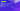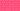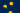Pricing# What is CAGR (compound annual growth rate)?

Written by

There are many different tools that you can use to compare investments and attempt to work out which is likely to provide you with higher returns. One of the most effective is the compound annual growth rate (CAGR), which provides you with a hypothetical constant growth rate for an investment or asset. But what is CAGR? Find out everything you need to know about the compound annual growth rate formula with our simple guide. First off, explore our CAGR definition.

## CAGR definition

So, what does CAGR stand for? Simple – compound annual growth rate. Essentially, CAGR is the measure of an asset or investment’s annual growth rate over a set period of time, while assuming compound growth. It’s important to remember that the compound annual growth rate formula doesn’t provide you with an actual return rate. Instead, it gives you a representational figure that describes the rate that your investment would have grown if it had grown at the same rate every year.

## Why is compound annual growth rate (CAGR) useful?

The compound annual growth rate (CAGR) has a broad number of uses. Most importantly, it can be used to measure and compare the performance of your company’s investments. CAGR assumes a constant growth rate across the period you’re looking at, which usually doesn’t reflect how investments work in reality. By smoothing volatile/inconsistent investment growth, it’s much easier to compare different investments or to track the performance of a specific investment.

## What is the compound annual growth rate formula?

You can work out CAGR using the following compound annual growth rate formula:

CAGR = [EV / BV]^1/n – 1

Where:

• EV = Ending value

• BV = Beginning value

• n = Number of years

For example, imagine that your company invested \$10,000 in Investment Fund X for three years. If the year-end value of the investment for each year is as follows:

• Year 1 – \$8,000

• Year 2 – \$10,500

• Year 3 – \$14,000

You can use the compound annual growth rate formula to calculate CAGR like so:

CAGR = (\$14,000 / \$10,000)^1/3 – 1 = 0.117 = 11.87%

If you would prefer, there is a broad range of compound annual growth rate calculators available online. Rather than doing the calculation by hand, you could use this CAGR calculator from CAGRCalculator.net, for example.

## Limitations of compound annual growth rate (CAGR)

Although CAGR can be an incredibly useful tool, there are a couple of disadvantages that you should keep in mind.

One of the most important limitations of the formula is also one of its strengths. The compound annual growth rate formula calculates a smoothed growth rate that ignores any potential volatility. Given that returns on investments are usually uneven – while CAGR also doesn’t account for investors adding or withdrawing funds from the portfolio – compound annual growth rate can sometimes provide you with an inflated growth rate.

Ignoring volatility can be very dangerous in financial planning, so you should be wary of using the compound annual growth rate for anything beyond comparing/tracking the performance of your investments.

## CAGR vs. IRR: what’s the difference?

On the face of it, CAGR and IRR (internal rate of return) may look similar, but there’s a critical difference that it’s essential to understand. Although IRR also helps you to measure the performance of your investments, it’s much more flexible than CAGR. Furthermore, the compound annual growth rate formula is simple enough that you can calculate it by hand. For more complicated projects/investments, IRR is a more suitable evaluation tool.

## We can help

GoCardless helps you automate payment collection, cutting down on the amount of admin your team needs to deal with when chasing invoices. Find out how GoCardless can help you with ad hoc payments or recurring payments.

Found this article interesting? Take a look at how to calculate Value at Risk (VaR)

Interested in automating the way you get paid? GoCardless can help

Contact salesSales

Contact sales

+1(415) 523-2279

Support

help@gocardless.com